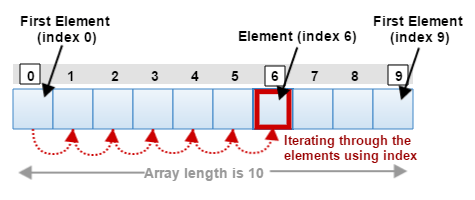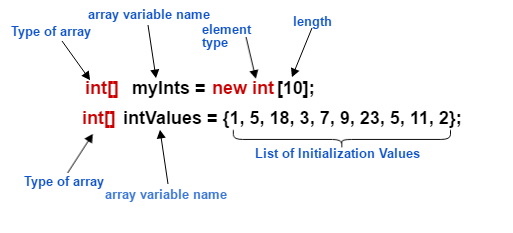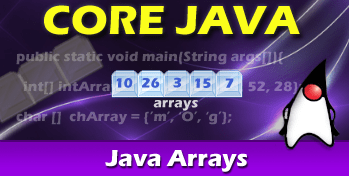# Java Tutorial – Java Arrays

## What is Array?

Java Arrays are containers that hold a fixed number of homogeneous elements. In other words, all of the data elements in the array are of the same data type. We define the length of the array when it is created. Each of the items in an array is called an element. These elements are each accessed by their numerical index beginning with index = 0.Say we have an array of 10 elements, we would have index range from 0 through 9.

## Declaring Array in Java

Declaring Java arrays follows the same conventions as when we declare variables of other types. We write the array as the type[]; the brackets [] are used to indicate that the variables holds an array. This is followed by the array’s name, which is whatever you like to call it, provided you follow standard naming conventions. For more on informaion on variable naming conventions, please refer to a previous post called, “Java Tutorial – Language Syntax and Structure”.

Declaring an array in Java has two formats; the developers have the option of using one of the following syntax:

#### Standard Convention

array_type[] array_name;
```int[] arrayOfInts;      // array of int primitives
long[] nationalDebt;    // array of long primitives
boolean[] isActive;     // array of boolean primitives
char[] arrayOfChars;    // array of char primitives
String[] arrayOfString; // array of String objects
```

or

#### Non-Standard Convention

array_type array_name[];
```short gamesPlayed[];  // array of short primitives
```

As you can see from the above examples, using the standard convention makes it easier to identify the array of a certain type when the brackets are next to the type assignment.

At this point, you will note that array size has not been defined. This means that the array array_name can be assigned any length. This will be explained shortly in the next section.

## Instantiating an Array in Java

When we declared the array previously, we actually did not create the array. We only instructed the Java compiler that the variable we declared will hold an array of a certain type. Instantiating an array happens when we use the new operator.

new type[size];
```int[] arrayOfInts;
char[] arrayOfChars;
String[] arrayOfString;

arrayOfInts = new int;
arrayOfChars = new char;
arrayOfString = new String;

arrayOfString = "Amaury Valdes";
arrayOfString = "Stacy Wilder";
arrayOfString = "Jane Hoffman";
...
```

In this example, we create three separate arrays. The first example creates an array of int 20 primitives. These primitives are all created with the default value of 0 (for int). The second array is created with a size of 100, and will be defaulted to ‘\u0000’ (for char). The final array of type String is created with a size of 100, and will be defaulted to null (for String).

We reference array elements by using an index. Please note that for arrays of size n, the valid indexes are between 0 and n-1.We can alternately declare and instantiate (create) arrays in one line.

```int[] arrayOfInts = new int;
char[] arrayOfChars = new char;
String[] arrayOfString = new String;
```

Be careful when referencing arrays using the index as using a negative number or a number greater than the array size will generate a java.lang.ArrayIndexOutOfBoundsException.

### Negative Array Size Exception

The java.lang.NegativeArraySizeException is an exception you will rarely see as it will only occur if you accidentally instantiate an array with an array size of negative number.

This may occur if the developer, for example, were assigning the size based on some computational calculation and the value became nagative.

```int[] arrayOfInts = new int[-17];
```

If you happen to put a negative size when you specify the array size during array creation you code will compile fine, but will generate a java.lang.NegativeArraySizeException during runtime.

## Initializing Array Literals

Java provides a mechanism of declaring, instantiating and explicitly initializing an array in one statement:

array_type[] array_name = { initialization list };
```int[] arrayOfInts = {1, 5, 18, 3, 7, 9, 23, 5, 11, 2};
```

As you can see from the above example, we can create and initialize an array without ever using the new keyword. Now, let look at how we can do the same for a String array.

```String[] names = {"Amaury Valdes", "Kim Lee", "Jane Ma"};
```

In this example, we create an array of three Strings and assign the array to the variable arrayOfString.

## Accessing Array Elements in Java

Each element in the array may be accessed through its numerical index. Using the examples above we can see how this would be achieved. In the first example, we access the second element in the names array by using index value of 1.

```logger("The Second Element in names array is " + names);
```

### Output

```The Second Element in names array is Kim Lee
```

In the next example, we access elements from the arrayOfInts array. Here we access the first, fifth, seventh, and last elements in the array by using the indexes of 0, 4, 6, and 9 respectively.

```logger("The First Element in arrayOfInts is " + arrayOfInts);
logger("The Fifth Element in arrayOfInts is " + arrayOfInts);
logger("The Seventh Element in arrayOfInts is " + arrayOfInts);
logger("The Last Element in arrayOfInts is " + arrayOfInts);
```

### Output

```The First Element in arrayOfInts is 0
The Fifth Element in arrayOfInts is 7
The Seventh Element in arrayOfInts is 23
The Last Element in arrayOfInts is 2
```

## Iterating through an Array

We will use the for loop and iterate over all of the elements

```int total = 0;
for (int i = 0; i < 10; i++) {
total = total + elem;
}
```

### Iterating through an Array with Enhanced Loop

The enhanced for-loop is a popular feature introduced in Java 5 Standard Edition. Using this new for-loop we simplify our code because we do not specify how to explicitly traverse all of the elements in the array. As we loop through our arrayOfInts array our elem variable will contain each element in the array.

By using the enhanced for-loop, we do not need to worry about bounds checking as there is no way that we can get java.lang.ArrayIndexOutOfBoundsException.

```int total = 0;
for (int elem: arrayOfInts) {
total = total + elem;
}
```

## Getting Array Length

We can get the number of the elements in an array by using the length property.

```int len = arrayOfInts.length;
logger("The length of arrayOfInts is " + len);
```

### Output

```The length of arrayOfInts is 10
```